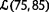# Table 2 Effects of $$\tilde {\beta }$$’s uncertain degrees on the prices under the three structures
$$\tilde {\beta }$$ w DD r DD p DD $$w_{d}^{DI}$$ $$r_{d}^{DI}$$ $$p_{d}^{DI}$$ $$p_{i}^{ID}$$ p II10.7648 7.0642 17.8289 8.1780 8.2140 16.3920 17.3695 16.000010.7607 7.0880 17.8487 8.1929 8.2371 16.4300 17.4173 16.045510.7567 7.1118 17.8685 8.2078 8.2602 16.4680 17.4651 16.090910.7527 7.1356 17.8883 8.2228 8.2833 16.5061 17.5129 16.1364